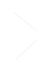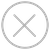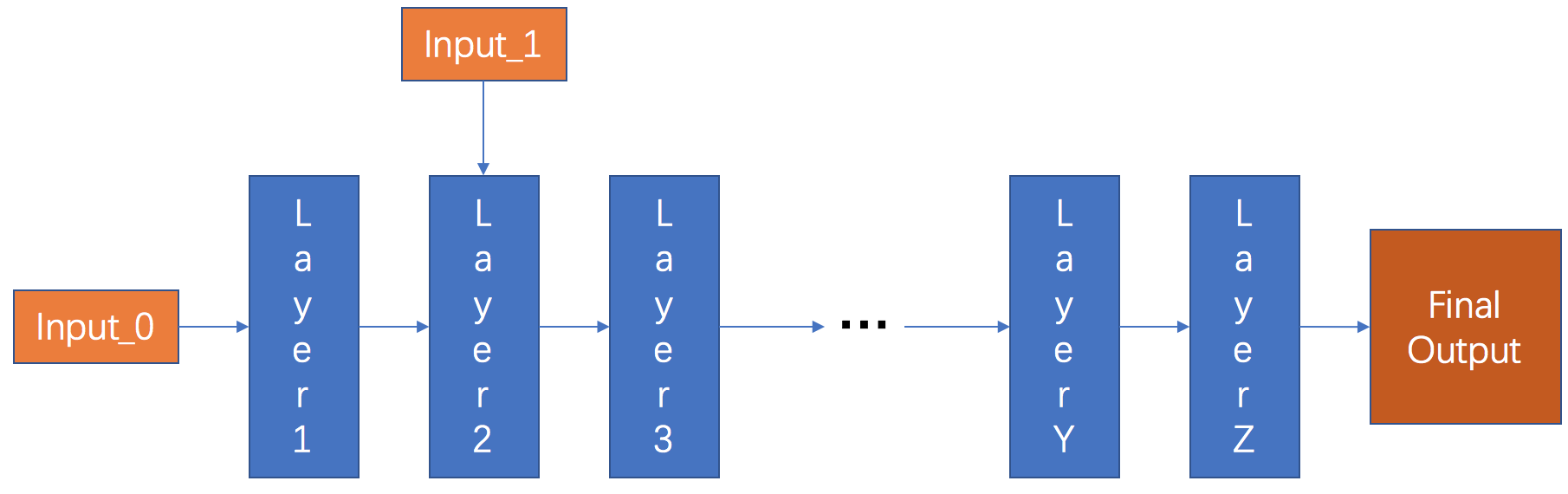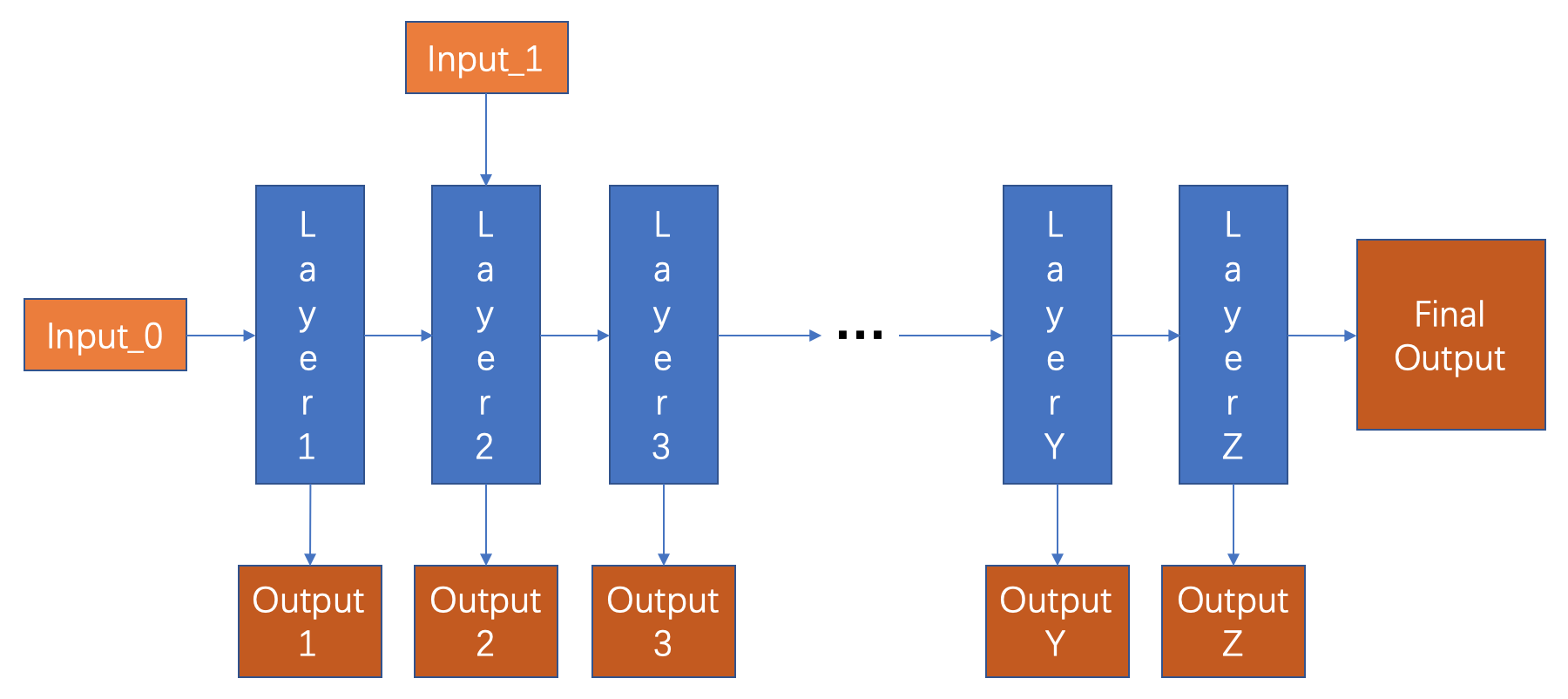TensorSpace.js
Getting StartBasic ConceptsTensorSpace ConverterModel PreprocessingModelsLayersMerge FunctionPreprocessing Introduction
It is confusing for the new TensorSpace developer to preprocess the pre-trained models: "What is a model preprocessing?", "Why do we need to preprocess the model?" and " How can we make it?". Then this introduction should somehow help you to understand the preprocessing.
What is a model preprocessing?
A model preprocessing for TensorSpace is the process to detect necessary data (intermediate layers/tensors), extract intermediate outputs from hidden layers and convert to TensorSpace compatible tfjs model format.
Why do we need a model preprocessing?
Typically, the trained model consumes the input data from the users and then computes among different layers/tensors and finally returns the meaningful outputs which can be used for further evaluations.Fig. 1 - Classic pre-trained model with single output
TensorSpace is a flexible library: we can construct a model without any existed network or trained weights to show the general structure of the model. It is intuitive to design and explain the prototype of a network before any construction and training.
However, the beauties of TensorSpace as a 3D data visualization model are not only about showing the model structure - how to construct a network, but also about presenting the data interactions among different intermediate layers - how to generate the final outputs step by step.
Hence, we need to find a way to collect the intermediate outputs from not only the last few output layers, but also from the intermediate hidden layers.Fig. 2 - TensorSpace compatible model with intermediate outputs
How do we preprocess a model?
To fully apply the core functionality of TensorSpace, we need to transfer the classic model (only returns the final output) into a new model (generates all intermediate outputs we want to present). For the following sections, we introduce how to use TensorFlow-Converter to preprocess and use TensorSpace to visualize the preprocessed models built by TensorFlow, Keras, and TensorFlow.js.